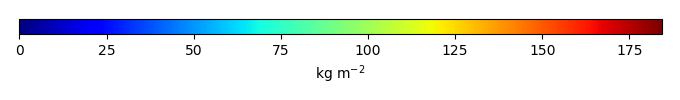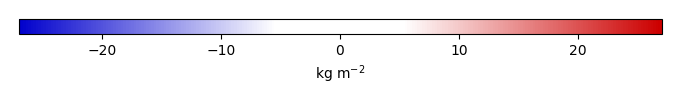# Mean State

Period Mean (original grids) [Pg]
Model Period Mean (intersection) [Pg]
Model Period Mean (complement) [Pg]
Benchmark Period Mean (intersection) [Pg]
Benchmark Period Mean (complement) [Pg]
Bias [kg m-2]
Bias Score 
Spatial Distribution Score 
Overall Score 
Benchmark [-] 34.1
CRUNCEPv7 [-] 41.4 42.1 0.279 33.8 0.315 2.30 0.62 0.80 0.71
GSWP3v1 [-] 30.5 30.9 0.269 33.8 0.315 -0.226 0.73 0.95 0.84
WATCH [-] 25.6 26.2 0.188 33.8 0.315 -1.35 0.74 0.86 0.80
Period Mean (original grids) [Pg]
Model Period Mean (intersection) [Pg]
Model Period Mean (complement) [Pg]
Benchmark Period Mean (intersection) [Pg]
Benchmark Period Mean (complement) [Pg]
Bias [kg m-2]
Bias Score 
Spatial Distribution Score 
Overall Score 
Benchmark [-] 44.8
CRUNCEPv7 [-] 17.5 16.5 0.0170 44.4 0.478 -4.09 0.51 0.85 0.68
GSWP3v1 [-] 15.3 14.4 0.0241 44.4 0.478 -4.37 0.50 0.78 0.64
WATCH [-] 17.4 16.5 0.0314 44.4 0.478 -4.07 0.53 0.82 0.67
Period Mean (original grids) [Pg]
Model Period Mean (intersection) [Pg]
Model Period Mean (complement) [Pg]
Benchmark Period Mean (intersection) [Pg]
Benchmark Period Mean (complement) [Pg]
Bias [kg m-2]
Bias Score 
Spatial Distribution Score 
Overall Score 
Benchmark [-] 60.1
CRUNCEPv7 [-] 40.1 38.8 0.989 59.3 0.762 -1.70 0.59 0.79 0.69
GSWP3v1 [-] 28.7 27.8 0.749 59.3 0.762 -2.94 0.55 0.84 0.69
WATCH [-] 32.4 31.6 0.679 59.3 0.762 -2.57 0.59 0.88 0.74
Period Mean (original grids) [Pg]
Model Period Mean (intersection) [Pg]
Model Period Mean (complement) [Pg]
Benchmark Period Mean (intersection) [Pg]
Benchmark Period Mean (complement) [Pg]
Bias [kg m-2]
Bias Score 
Spatial Distribution Score 
Overall Score 
Benchmark [-] 49.3
CRUNCEPv7 [-] 248. 246. 2.31 48.3 0.997 62.9 0.22 0.031 0.13
GSWP3v1 [-] 101. 99.2 1.16 48.3 0.997 17.5 0.43 0.12 0.28
WATCH [-] 127. 125. 1.66 48.3 0.997 26.3 0.33 0.11 0.22
Period Mean (original grids) [Pg]
Model Period Mean (intersection) [Pg]
Model Period Mean (complement) [Pg]
Benchmark Period Mean (intersection) [Pg]
Benchmark Period Mean (complement) [Pg]
Bias [kg m-2]
Bias Score 
Spatial Distribution Score 
Overall Score 
Benchmark [-] 55.7
CRUNCEPv7 [-] 25.3 23.5 1.53 50.7 4.99 -4.37 0.69 0.30 0.50
GSWP3v1 [-] 20.6 19.1 1.31 50.7 4.99 -6.12 0.65 0.27 0.46
WATCH [-] 18.4 17.1 1.18 50.7 4.99 -7.06 0.62 0.22 0.42
Period Mean (original grids) [Pg]
Model Period Mean (intersection) [Pg]
Model Period Mean (complement) [Pg]
Benchmark Period Mean (intersection) [Pg]
Benchmark Period Mean (complement) [Pg]
Bias [kg m-2]
Bias Score 
Spatial Distribution Score 
Overall Score 
Benchmark [-] 131.
CRUNCEPv7 [-] 294. 288. 0.706 130. 0.462 17.5 0.49 0.33 0.41
GSWP3v1 [-] 133. 130. 0.561 130. 0.462 0.0856 0.64 0.88 0.76
WATCH [-] 137. 133. 0.501 130. 0.462 0.439 0.64 0.89 0.76
Period Mean (original grids) [Pg]
Model Period Mean (intersection) [Pg]
Model Period Mean (complement) [Pg]
Benchmark Period Mean (intersection) [Pg]
Benchmark Period Mean (complement) [Pg]
Bias [kg m-2]
Bias Score 
Spatial Distribution Score 
Overall Score 
Benchmark [-] 153.
CRUNCEPv7 [-] 122. 125. 0.725 151. 2.09 -0.996 0.65 0.56 0.61
GSWP3v1 [-] 91.8 93.6 0.579 151. 2.09 -2.83 0.62 0.90 0.76
WATCH [-] 115. 117. 0.571 151. 2.09 -1.59 0.61 0.71 0.66
Period Mean (original grids) [Pg]
Model Period Mean (intersection) [Pg]
Model Period Mean (complement) [Pg]
Benchmark Period Mean (intersection) [Pg]
Benchmark Period Mean (complement) [Pg]
Bias [kg m-2]
Bias Score 
Spatial Distribution Score 
Overall Score 
Benchmark [-] 58.4
CRUNCEPv7 [-] 37.4 35.7 0.948 56.8 1.57 -1.91 0.63 0.77 0.70
GSWP3v1 [-] 33.0 31.1 0.855 56.8 1.57 -2.67 0.66 0.86 0.76
WATCH [-] 31.0 29.6 0.759 56.8 1.57 -2.95 0.66 0.93 0.80
Period Mean (original grids) [Pg]
Model Period Mean (intersection) [Pg]
Model Period Mean (complement) [Pg]
Benchmark Period Mean (intersection) [Pg]
Benchmark Period Mean (complement) [Pg]
Bias [kg m-2]
Bias Score 
Spatial Distribution Score 
Overall Score 
Benchmark [-] 59.4
CRUNCEPv7 [-] 254. 256. 1.05 58.9 0.523 56.8 0.34 0.055 0.20
GSWP3v1 [-] 119. 120. 0.656 58.9 0.523 18.1 0.45 0.23 0.34
WATCH [-] 84.2 84.4 0.890 58.9 0.523 8.25 0.49 0.40 0.45
Period Mean (original grids) [Pg]
Model Period Mean (intersection) [Pg]
Model Period Mean (complement) [Pg]
Benchmark Period Mean (intersection) [Pg]
Benchmark Period Mean (complement) [Pg]
Bias [kg m-2]
Bias Score 
Spatial Distribution Score 
Overall Score 
Benchmark [-] 58.9
CRUNCEPv7 [-] 30.2 28.1 1.77 57.4 1.53 -2.42 0.53 0.38 0.45
GSWP3v1 [-] 27.6 25.8 1.60 57.4 1.53 -2.75 0.54 0.47 0.51
WATCH [-] 25.8 24.4 1.31 57.4 1.53 -3.03 0.55 0.56 0.55
Period Mean (original grids) [Pg]
Model Period Mean (intersection) [Pg]
Model Period Mean (complement) [Pg]
Benchmark Period Mean (intersection) [Pg]
Benchmark Period Mean (complement) [Pg]
Bias [kg m-2]
Bias Score 
Spatial Distribution Score 
Overall Score 
Benchmark [-] 66.2
CRUNCEPv7 [-] 67.9 65.8 1.17 64.6 1.58 2.15 0.70 0.95 0.82
GSWP3v1 [-] 44.2 42.7 0.830 64.6 1.58 -2.44 0.72 0.85 0.78
WATCH [-] 33.7 32.5 0.752 64.6 1.58 -4.47 0.65 0.63 0.64
Period Mean (original grids) [Pg]
Model Period Mean (intersection) [Pg]
Model Period Mean (complement) [Pg]
Benchmark Period Mean (intersection) [Pg]
Benchmark Period Mean (complement) [Pg]
Bias [kg m-2]
Bias Score 
Spatial Distribution Score 
Overall Score 
Benchmark [-] 1.38e+03
CRUNCEPv7 [-] 1.92e+03 1.90e+03 22.7 1.32e+03 56.4 5.01 0.56 0.10 0.33
GSWP3v1 [-] 1.06e+03 1.04e+03 16.5 1.32e+03 56.4 -1.32 0.60 0.43 0.51
WATCH [-] 1.09e+03 1.07e+03 17.3 1.32e+03 56.4 -1.10 0.60 0.34 0.47
Period Mean (original grids) [Pg]
Model Period Mean (intersection) [Pg]
Model Period Mean (complement) [Pg]
Benchmark Period Mean (intersection) [Pg]
Benchmark Period Mean (complement) [Pg]
Bias [kg m-2]
Bias Score 
Spatial Distribution Score 
Overall Score 
Benchmark [-] 64.3
CRUNCEPv7 [-] 18.3 18.0 0.405 63.6 0.726 -2.65 0.50 0.76 0.63
GSWP3v1 [-] 14.0 13.8 0.386 63.6 0.726 -2.91 0.48 0.82 0.65
WATCH [-] 15.6 15.4 0.362 63.6 0.726 -2.83 0.49 0.81 0.65
Period Mean (original grids) [Pg]
Model Period Mean (intersection) [Pg]
Model Period Mean (complement) [Pg]
Benchmark Period Mean (intersection) [Pg]
Benchmark Period Mean (complement) [Pg]
Bias [kg m-2]
Bias Score 
Spatial Distribution Score 
Overall Score 
Benchmark [-] 90.9
CRUNCEPv7 [-] 326. 324. 2.83 89.5 1.44 35.8 0.33 0.077 0.20
GSWP3v1 [-] 123. 122. 1.25 89.5 1.44 5.76 0.44 0.42 0.43
WATCH [-] 154. 151. 2.16 89.5 1.44 10.5 0.48 0.17 0.33
Period Mean (original grids) [Pg]
Model Period Mean (intersection) [Pg]
Model Period Mean (complement) [Pg]
Benchmark Period Mean (intersection) [Pg]
Benchmark Period Mean (complement) [Pg]
Bias [kg m-2]
Bias Score 
Spatial Distribution Score 
Overall Score 
Benchmark [-] 42.5
CRUNCEPv7 [-] 21.0 19.3 1.14 40.2 2.26 -3.97 0.57 0.84 0.70
GSWP3v1 [-] 16.9 15.5 0.942 40.2 2.26 -5.56 0.57 0.73 0.65
WATCH [-] 15.4 14.3 0.741 40.2 2.26 -6.18 0.56 0.65 0.61
Period Mean (original grids) [Pg]
Model Period Mean (intersection) [Pg]
Model Period Mean (complement) [Pg]
Benchmark Period Mean (intersection) [Pg]
Benchmark Period Mean (complement) [Pg]
Bias [kg m-2]
Bias Score 
Spatial Distribution Score 
Overall Score 
Benchmark [-] 45.4
CRUNCEPv7 [-] 42.8 41.7 0.382 44.5 0.888 -0.108 0.75 0.84 0.79
GSWP3v1 [-] 32.2 31.2 0.308 44.5 0.888 -2.77 0.72 0.77 0.75
WATCH [-] 31.5 30.6 0.255 44.5 0.888 -2.98 0.72 0.71 0.72
Period Mean (original grids) [Pg]
Model Period Mean (intersection) [Pg]
Model Period Mean (complement) [Pg]
Benchmark Period Mean (intersection) [Pg]
Benchmark Period Mean (complement) [Pg]
Bias [kg m-2]
Bias Score 
Spatial Distribution Score 
Overall Score 
Benchmark [-] 88.1
CRUNCEPv7 [-] 145. 143. 0.333 86.3 1.76 11.0 0.58 0.47 0.53
GSWP3v1 [-] 75.2 75.3 0.272 86.3 1.76 -1.69 0.63 0.87 0.75
WATCH [-] 68.6 68.8 0.269 86.3 1.76 -2.94 0.63 0.84 0.73
Period Mean (original grids) [Pg]
Model Period Mean (intersection) [Pg]
Model Period Mean (complement) [Pg]
Benchmark Period Mean (intersection) [Pg]
Benchmark Period Mean (complement) [Pg]
Bias [kg m-2]
Bias Score 
Spatial Distribution Score 
Overall Score 
Benchmark [-] 34.9
CRUNCEPv7 [-] 12.9 13.5 0.124 34.7 0.216 -5.04 0.55 0.83 0.69
GSWP3v1 [-] 11.5 12.1 0.0886 34.7 0.216 -5.41 0.54 0.87 0.71
WATCH [-] 12.5 13.1 0.0918 34.7 0.216 -5.19 0.54 0.86 0.70
Period Mean (original grids) [Pg]
Model Period Mean (intersection) [Pg]
Model Period Mean (complement) [Pg]
Benchmark Period Mean (intersection) [Pg]
Benchmark Period Mean (complement) [Pg]
Bias [kg m-2]
Bias Score 
Spatial Distribution Score 
Overall Score 
Benchmark [-] 101.
CRUNCEPv7 [-] 86.4 85.4 0.425 100. 0.811 -1.19 0.73 0.59 0.66
GSWP3v1 [-] 68.5 67.8 0.372 100. 0.811 -3.35 0.69 0.46 0.58
WATCH [-] 69.1 68.4 0.317 100. 0.811 -3.30 0.71 0.33 0.52
Period Mean (original grids) [Pg]
Model Period Mean (intersection) [Pg]
Model Period Mean (complement) [Pg]
Benchmark Period Mean (intersection) [Pg]
Benchmark Period Mean (complement) [Pg]
Bias [kg m-2]
Bias Score 
Spatial Distribution Score 
Overall Score 
Benchmark [-] 60.7
CRUNCEPv7 [-] 62.6 63.0 0.653 60.4 0.300 1.03 0.65 0.91 0.78
GSWP3v1 [-] 47.2 47.5 0.479 60.4 0.300 -1.44 0.67 0.84 0.75
WATCH [-] 48.4 48.9 0.413 60.4 0.300 -1.30 0.69 0.80 0.75
Period Mean (original grids) [Pg]
Model Period Mean (intersection) [Pg]
Model Period Mean (complement) [Pg]
Benchmark Period Mean (intersection) [Pg]
Benchmark Period Mean (complement) [Pg]
Bias [kg m-2]
Bias Score 
Spatial Distribution Score 
Overall Score 
Benchmark [-] 29.6
CRUNCEPv7 [-] 17.7 16.8 0.278 28.2 1.46 -2.29 0.56 0.73 0.64
GSWP3v1 [-] 15.7 14.8 0.272 28.2 1.46 -3.23 0.57 0.49 0.53
WATCH [-] 15.3 14.5 0.227 28.2 1.46 -3.44 0.56 0.51 0.54

# Temporally integrated period mean

BENCHMARK MEANMODEL MEANBIASBIAS SCORESPATIAL TAYLOR DIAGRAMMODEL COLORS# Spatially integrated regional mean

MODEL COLORS# All Models

BenchmarkCRUNCEPv7GSWP3v1WATCH# Data Information

Approach: I just read the data from the original data file and flip the latitude arrange from original 90N->90S to 90S->90N.

Temporal resolution: yearly

General information: This product was derived from Harmonized World Soil Database (HWSD) v1.2 dataset.

Spatial resolution: 0.5x0.5 degree